# Accelerator, Particle

Also found in: Dictionary, Thesaurus.

## Accelerator, Particle

a device that accelerates charged particles to high energies. The particles may be electrons, protons, atomic nuclei, or ions.

The acceleration is accomplished with an electric field, which is capable of altering the energy of particles that carry an electric charge. Since a magnetic field can change the direction of the motion of charged particles but not their speed, it is used in accelerators to control the shape of the particle trajectory. The accelerating electric field is usually generated by external devices. In what is called collective acceleration, however, charged particles are accelerated by means of fields produced by other charged particles (see). Particle accelerators should be distinguished from plasma accelerators, which produce charged-particle fluxes that, on the average, are electrically neutral.

Particle accelerators are one of the basic tools of modern physics. They are used to provide beams of primary accelerated charged particles and beams of the secondary particles—such as mesons, neutrons, and photons—that are produced when primary accelerated particles interact with matter. High-energy particle beams are employed in the study of the nature and properties of elementary particles and find application in nuclear and solid state physics. They are also coming into increasing use in research in such fields as chemistry, biophysics, and geophysics. The importance of particle accelerators in various energy ranges is growing in other areas as well. In metallurgy, for example, accelerators are used to detect flaws in parts and structures. The wood-products industry employs accelerators to carry out rapid high-quality working of articles. In the food-processing industry accelerators are used to sterilize food products. In medicine accelerators find application in radiotherapy and “bloodless surgery.”

The development of particle accelerators was stimulated by the need for fluxes of high-energy charged particles to carry out investigations of the structure of the atomic nucleus. Natural charged-particle sources, that is, radioactive elements, were originally used, but they were limited both in the intensity and the energy of the particles emitted. In 1919, E. Rutherford used a flux of alpha particles from a radioactive source to produce the first artificial transmutation of nuclei. This achievement gave rise to a search for methods of producing beams of accelerated particles.

In the initial period of accelerator development, which lasted from 1919 to 1932, efforts were concentrated on the obtaining of high voltages and the use of such voltages to carry out the direct acceleration of charged particles. The American physicist R. Van de Graaff built an electrostatic generator in 1931, and the British physicists J. Cockcroft and E. Walton of Rutherford’s laboratory developed a type of cascade generator in 1932. These devices made it possible to produce fluxes of accelerated particles with an energy of the order of 1 million electron volts (MeV). A nuclear reaction induced by artifically accelerated particles—the splitting of the lithium nucleus by protons—was accomplished for the first time in 1932.

The years from 1931 to 1944 were a period of the inception and development of the resonance method of acceleration. In this method the particles being accelerated pass many times through an accelerating gap; they thus can acquire a high energy even when a moderate accelerating voltage is used. The cyclotron, a circular resonance accelerator invented by E. O. Lawrence, soon surpassed electrostatic accelerators in particle energy. By the end of the period a proton energy of the order of 10–20 MeV had been achieved in cyclotrons. Although resonance acceleration is also possible in linear accelerators, linear resonance accelerators did not come into use in this period because of the inadequate development of radio engineering. In 1940 the American physicist D. W. Kerst built a circular induction accelerator, called the betatron, for electrons; the idea of such an accelerator had been advanced by the American physicist J. Slepian in 1922 and the Swiss physicist R. Wideröe in 1928.

The development of phase-stable, or synchronous, accelerators began in 1944, when the Soviet physicist V. I. Veksler discovered the principle of phase stability; the principle was independently discovered slightly later by the American physicist E. M. McMillan. The application of the phase stability principle to resonance accelerators makes possible a considerable increase in the energy of the accelerated particles. Such new types of resonance accelerators as the electron synchrotron, synchrocyclotron, proton synchrotron, and microtron were proposed on the basis of the principle. At the same time, advances in radio engineering made possible the building of efficient linear resonance accelerators of electrons and heavy charged particles.

The principle of alternating-gradient focusing of particles was proposed by the Greek engineer N. Christofilos in 1950 and the American scientists E. Courant, M. Livingston, and H. Snyder in 1952. Alternating-gradient focusing permitted a substantial increase in the upper limit of the energies attainable in circular and linear accelerators. In 1956, Veksler published a paper in which he advanced the notion of the coherent, or collective, method of particle acceleration.

The following two decades saw the realization of the ideas mentioned above and the achievement of technical improvements in particle accelerators. Linear resonance accelerators proved to be the most promising machines for electron acceleration. The largest such accelerator went into operation at Stanford University in the USA in 1966. Constructed under the direction of the American physicist W. Panofsky, it is capable of accelerating electrons to an energy of 22 gigaelectron volts (GeV). The highest proton energies have been achieved in proton synchrotrons. In 1957 a 10-GeV proton synchrotron, which at the time was the largest in the world, went into operation at Dubna in the USSR. A few years later, alternating-gradient proton synchrotrons with energies of 25–30 GeV were completed in Switzerland and the USA. In 1967 a 76-GeV proton synchrotron went into operation near Serpukhov in the USSR; it was for several years the largest in the world. A 200-GeV proton synchrotron was completed in the USA in 1972; design improvements enabled it to reach 400 GeV. Work on accelerators for the energy range from 1,000 to 5,000 GeV is at various stages of design or construction in the USSR and the USA.

The lines of development of particle accelerators at the present time include increasing the energy of the accelerated particles, increasing the intensity (current) and pulse duration of the accelerated beam, and improving beam quality (reducing the spread in energy, transverse coordinates, and velocities). Along with the development of new acceleration methods, improvements are being made in traditional methods. For example, work is being carried out on the use of superconducting materials and associated low-temperature equipment in magnets and accelerating systems in order to reduce sharply power consumption and the dimensions of magnet systems. The range of application of automatic control methods in accelerators is being expanded. Accelerators are being supplemented with storage rings that permit the investigation of elementary interactions in colliding beams (see). In improving traditional acceleration methods, particular attention is paid to reducing the cost of the accelerators.

Particle accelerators may be classified in different ways. With respect, for example, to the type of particles being accelerated, electron accelerators, proton accelerators, and ion accelerators are distinguished.

With respect to the character of the particle trajectories, a distinction is made between linear accelerators and circular, or cyclic, accelerators. In linear accelerators, which are also known as linacs, the particle trajectories are close to a straight line; in circular accelerators the particle trajectories are close to a circle or spiral.

Depending on the character of the accelerating field, particle accelerators are divided into resonance and nonresonance accelerators. In resonance machines acceleration is accomplished with an alternating radio-frequency electromagnetic field; for successful acceleration to occur, the particles must move in resonance with the field. In nonresonance accelerators the direction of the field does not change during acceleration. Nonresonance machines are divided into induction accelerators and high-voltage accelerators. In induction accelerators the accelerating electric field is generated through a change in the magnetic field: the particles are accelerated by the induced electromotive force. In high-voltage accelerators the accelerating field is due to a directly applied potential difference.

Depending on the focusing mechanism, which ensures stability of particle motion in directions perpendicular to the orbit, a distinction is made between constant-gradient, or weak-focusing,

Table 1. Classification of circular accelerators (accelerators with circular or spiral-like particle trajectories)
Character of accelerating fieldMagnetic guide fieldFrequency of accelerating fieldFocusingType of acceleratorParticles accelerated
Nonresonance, inductionVariableConstant-gradientBetatronElectrons
ResonanceConstantConstantConstant-gradientCyclotronProtons or ions
ResonanceConstantConstantConstant-gradientMicrotronElectrons
ResonanceConstantConstantAlternating-gradientIsochronous cyclotronProtons
ResonanceConstantConstantAlternating-gradientSector microtronElectrons
ResonanceConstantVariableConstant-gradientSynchrocyclotronProtons
ResonanceConstantVariableAlternating-gradientSector synchrocyclotronProtons
ResonanceVariableConstantConstant-gradientWeak-focusing electron synchrotronElectrons
ResonanceVariableConstantAlternating-gradientAlternating-gradient electron synchrotronElectrons
ResonanceVariableVariableConstant-gradientWeak-focusing protron synchrotronProtons
ResonanceVariableVariableAlternating-gradientAlternating-gradient protron synchrotronProtons
Table 2. Classification of linear accelerators (accelerators with straight-line particle trajectories)
Character of accelerating fieldFrequency of accelerating fieldType of acceleratorParticles accelerated
Nonresonance, electrostaticElectrostatic accelerator, cascade acceleratorProtons, electrons
Nonresonance, inductionLinear induction acceleratorElectrons
ResonanceConstantLinear resonance acceleratorProtons, electrons

accelerators, in which the focusing force is constant along the trajectory (at least with respect to sign), and alternating-gradient, or strong-focusing, accelerators, in which the focusing force changes sign along the trajectory—that is, focusing and defocusing sections alternate.

Circular resonance accelerators may be further classified according to the character of the controlling magnetic field (the guide field) and the accelerating electric field. The magnetic field may be constant or vary with time, and the frequency of the accelerating field may be constant or vary with time.

The classification of particle accelerators is summarized in Tables 1 and 2. Two types of machines are not encompassed by the given classification: colliding-beam machines and accelerators that make use of collective acceleration methods. Colliding-beam machines represent a distinctive modification of the accelerators listed in Tables 1 and 2: particle beams from an accelerator of a given type are directed against each other so as to collide head on. Collective-acceleration machines differ from all the described accelerators with respect to the source of the accelerating field.

In a resonance accelerator a particle is continually accelerated, since it always crosses the accelerating gap at an accelerating phase of the field—that is, when the electric field is directed with the particle motion. An ideal, or equilibrium, particle always crosses the gap at the same, equilibrium phase.

In a circular accelerator the relation between a particle’s period T of revolution and the average radius <R> of the particle’s orbit is given by the equation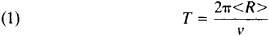where v is the speed of the particle. For the average orbit radius we haveHere, E = mc2 is the total relativistic energy of a particle of mass m (this energy is equal to the sum of the particle’s rest energy E0= m0c2 and its kinetic energy W, where m0 is the particle’s rest mass and c is the speed of light), e is the charge of the particle, and <B> is the average value of the magnetic induction. Consequently, the relation between the period of revolution and the particle’s energy is given by the equation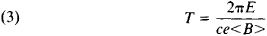For an equilibrium particle the period of revolution is equal to or a multiple of the period Ta of the accelerating field.

To fixed values of the revolution period and magnetic induction there correspond a definite equilibrium energy of the particle and equilibrium radius of its orbit. An equilibrium particle acquires in one revolution the energy eV0 cos φ0, where φ0 is the equilibrium phase (that is, the phase of the field acting on an equilibrium particle as reckoned from the field maximum) and V0 is the voltage amplitude at the gap of the accelerating electrodes. To acquire a final kinetic energy Wmax, a particle must complete N = Wmax/eV0 cos φ0 revolutions.

The distance traversed by a particle in a circular accelerator can reach tens or hundreds of thousands of kilometers. With such great path lengths, successful operation of the accelerator requires stability of equilibrium motion: small deviations of the particle with respect to phase or energy, small radial or vertical excursions of the particle, and small initial speeds in directions perpendicular to the orbit must not lead to significant departure of the particle from the equilibrium orbit. In other words, the particle must undergo an oscillatory motion about the equilibrium particle. The stability of particle motion in directions perpendicular to the orbit (the radial and vertical directions) is provided by focusing. Stability in the direction of the orbit is provided by the phase stability mechanism (phase focusing).

In a proton linear accelerator with accelerating gaps the time required for an equilibrium particle to travel between adjacent accelerating gaps is T = L/v, where L is the distance between the centers of the gaps and v is the particle speed. T is a multiple of the period of the accelerating field Ta = λ/c, where λ is the wavelength of the electromagnetic field. An energy Wmax is acquired upon passage through N = Wmax/eV0 cos φ0 accelerating gaps; this fact determines the required length of the accelerator. Since the lengths of present-day proton linear accelerators reach hundreds of meters, the problem of stability of particle motion arises; to deal with this problem, focusing and phase stability are made use of.

In order that scattering by the nuclei of gas atoms not cause particles to make substantial deviations from the equilibrium trajectory and to drop out of the acceleration process, the region around the equilibrium trajectory is enclosed by a vacuum chamber in which special pumps are used to produce a sufficiently high vacuum.

Phase focusing. Phase focusing in resonance accelerators is provided by the phase stability principle, which is associated with the energy dependence of the time interval between successive accelerations. In weak-focusing circular accelerators the period of revolution increases with increasing energy, since in equation (1) the average orbit radius increases more rapidly with increasing energy than does the particle speed. In alternating-gradient accelerators the energy dependence of the average orbit radius is much weaker. Consequently, at low energies the period of revolution usually decreases with an increase in energy (v increases faster than <R>), and at high energies the revolution period increases with increasing energy (<R> increases more rapidly than v, which is limited by the speed of light). For a period increasing with energy, the right-hand phase in Figure 1 is stable: if a particle reaches the accelerating gap at a phase φ1, > φ0, the particle will acquire an energy less than the equilibrium energy. Consequently, the period of revolution will become less than the equilibrium period, and the particle’s phase will shift so as to approach the equilibrium phase φ0. If, however, the period decreases with increasing energy, the phase φ0 is unstable, and the phase – φ0 symmetric to it is stable. In any case, if eV0 is sufficiently great, there always exists a stable equilibrium phase and a region of phases close to it within which the particles are trapped and oscillate about the equilibrium phase.Figure 1. Principle of phase stability

The increase in the energy of the equilibrium particle eV0 cos φ0 is determined by the resonance condition T = qTa, where q is an integer. For a circular accelerator the energy of the equilibrium particle is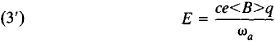where ωa = 2π/Ta is the accelerating-field frequency. Thus, the equilibrium energy can be increased by increasing the magnetic field (as in an electron synchrotron), by reducing the accelerating-field frequency (as in a synchrocyclotron), by changing both the magnetic field and the accelerating-field frequency (as in a proton synchrotron), or by varying q (as in a microtron). The law governing the variation of the magnetic field, frequency, and q determines the value of the phase φ0 for an equilibrium particle; because of phase stability, an equilibrium particle acquires precisely the energy given by equation (3’). The radius of the equilibrium orbit, which is given by equation (2), varies with energy.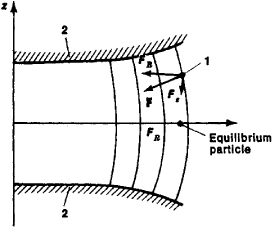Figure 2. In the presence of a “barrel-shaped” magnetic field, the magnetic force acting on a particle displaced from the equilibrium orbit has a vertically focusing component: (F) magnetic force acting on particle, (Fx) vertical component of F, (FR) radial component of F, (1) particle displaced from equilibrium orbit, (2) pole faces

For particles with a phase sufficiently close to that of an equilibrium particle, the increase in energy occurs nonuniformly, but, on the average, they acquire the same energy as the equilibrium particle. These particles are trapped in the acceleration regime. Particles that differ markedly from the equilibrium particle in phase or energy do not, on the average, acquire energy, since they are subjected sometimes to the accelerating field and sometimes to the decelerating field.

A similar mechanism of phase focusing operates in linear resonance accelerators. In this case, however, the time required to traverse the distances between adjacent gaps always decreases with increasing energy. The stable equilibrium phase thus is always – φ0.

Focusing. In circular accelerators focusing is achieved principally by special selection of the shape of the magnetic field. If the magnetic field were strictly uniform, then any deviation of particle velocity from the orbital plane would cause the particle being accelerated to depart from the equilibrium orbit in the direction of the axis of the magnet—that is, in the vertical, or z, direction. If, however, the magnetic field decreases with increasing radius, then it has a “barrel-like” shape, since in the absence of currents the magnetic field is irrotational; the force F acting on a particle consequently has a component Fz directed toward the plane of the equilibrium orbit (Figure 2).

The radial variation of the field commonly is characterized by the field index n = – ∂(ln B)/∂(ln R). Thus, for stability of motion in the vertical, or axial, direction the condition n > 0 must be satisfied—that is, the field must decrease with increasing radius. Motion in the radial direction is determined by the relation between the magnetic force eBv/c acting on the particle and the centripetal force mv2/R required to hold the particle in its orbit. In an equilibrium orbit these two quantities are equal. Should a particle with the same speed be displaced to a larger radius, to ensure radial stability the magnetic force eBvlc acting at this radius would have to be greater than mv2/R—that is, the magnetic field would have to decrease more slowly than 1/R. The same conclusion is obtained if a random displacement of the particle to a shorter radius is considered. The condition for radial stability thus imposes a limitation on the rate of decrease of the magnetic field: the field index n must be less than 1. For simultaneous stability in the radial and vertical directions, the following condition must be satisfied:

(4) 0 < n < 1

It can be shown that the radial and vertical focusing forces when this condition is fulfilled are

(5) FR = –(1 – n)mω2ΔR

Fz = – nmω2Δz

where m is the mass of the particle, ω is the angular frequency of the particle, and ΔR and Δz are the radial and vertical displacements of the particle from the equilibrium orbit. When these focusing forces operate, the particles undergo oscillations, called betatron oscillations, about the equilibrium orbit at frequenciesThese frequencies are less than ω—that is, in one orbit a particle completes less than one betatron oscillation. The focusing forces are limited by the maximum permissible values of n. Such focusing is called constant-gradient, or weak, focusing.

To increase the vertical focusing force, a strongly decreasing field must be applied (n >> 1). By contrast, to obtain a large radial focusing force, a field with large negative values of n (that is, a field that increases strongly along the radius) must be applied. These requirements cannot be fulfilled simultaneously. Under certain restrictions, however, they can be fulfilled alternately; in this way strong radial and vertical focusing can be provided. The principle of alternating-gradient focusing is based on this fact (Figure 3).

In alternating-gradient focusing the entire length of the equilibrium orbit is divided into a large number of equal sections in which magnets that provide alternate radial and vertical strong focusing are mounted. Given a certain relation among the field indexes, magnet length, and number of sections, such a system exhibits strong focusing in both transverse directions. This result can be explained physically by noting that a particle is farther from the equilibrium position in a focusing magnet than in a defocusing magnet, since the preceding defocusing magnet deflected the particle from the orbit. The action of the focusing magnets is therefore stronger than that of the defocusing magnets.

The frequency of particle oscillations under such focusing is considerably higher than the frequency of revolution, so that a particle undergoes several oscillations in a single orbit. Since an increase in focusing force leads to a decrease in the amplitude of the particle oscillations due to various driving factors, the transverse dimensions of the vacuum chamber and magnets can be decreased, and, consequently, the weight and cost of the machine can be reduced. Alternating-gradient, or strong, focusing is therefore used in all large high-energy circular accelerators.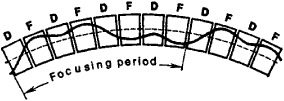Figure 3. Arrangement of magnets in an alternating-gradient accelerator: (D) magnets that defocus in the radial direction (n >> 1), (F) magnets that focus in the radial direction (n << –1). The broken line indicates the equilibrium orbit, and the solid line indicates the orbit of a particle displaced from the equilibrium orbit.

A drawback of strong focusing is the presence of numerous resonances due to the high frequency of the particle oscillations: if the number of vertical or radial oscillations of a particle in one complete orbit or the sum or difference thereof is an integer or half-integer, resonant buildup of the oscillations occurs. For this reason high demands must be placed on the precision of magnet fabrication.

Alternating-gradient focusing is also used in linear accelerators. In this case, however, the magnetic field in the equilibrium orbit, which is a straight line, is zero. The focusing system is a set of magnetic quadrupole lenses. The magnetic field they produce is zero at the axis O of the system and increases linearly with displacement from the axis (Figure 4). The magnets focus particles in one plane (the force F is directed toward the axis) and defocus in the other (F is directed away from the axis). The focusing planes alternate from magnet to magnet, so that alternating-gradient focusing results.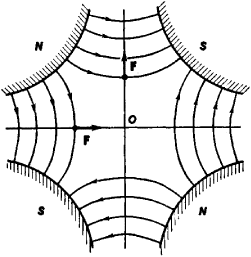Figure 4. Field of a magnetic quadrupole lens: (N) north poles of magnet, (S) south poles of magnet, (F) force exerted by magnetic field on a particle moving in a direction perpendicular to the plane of the figure. At the center O, F = 0.

At low particle energies, electric field focusing is employed in addition to magnetic focusing in both circular and linear accelerators. For this purpose the accelerating electric field of the machine is made use of. The focusing principle is illustrated in Figure 5. In a conventional accelerating gap the electric field usually “sags” inward at the center of the gap. In the first half of the gap the field therefore has a focusing component directed toward the axis of the gap, and in the second half it has a defocusing component directed away from the axis of the gap. A focusing effect results if the focusing action is greater than the defocusing action. Since a particle is accelerated when it crosses the gap, it moves more rapidly, and spends less time, in the second half of the gap than in the first half. Consequently, the focusing action predominates. This effect, which is based on the change in the particle’s speed, is sometimes called electrostatic focusing. It has an appreciable magnitude only for low particle speeds, and its use in accelerators therefore is limited. The difference between the action of the electric field in the first and second halves of the gap may also be due to the time variation of the electric field (electrodynamic focusing): if the electric field decreases as the particle passes through it, the defocusing action is less than the focusing action. Focusing of this type occurs in the cyclotron and synchrocyclotron as an additional factor to magnetic focusing. In linear proton accelerators, however, the negative phase φ0 (see above) is stable. At this phase the field increases with time. The electric field in linear accelerators therefore defocuses, and special additional measures are required for particle focusing.Figure 5. Distribution of the electric field in the accelerating gap between two electrodes: (A) and (B) electrodes, (F) force acting on particle, (Fx) longitudinal component of F, (Fy) transverse component of F

The principle of alternating-gradient focusing can be applied to an electric field. For example, by using electrodes of complex shape it is possible to effect a change in the sign of the focusing force from gap to gap. Moreover, by also changing the sign of the equilibrium phase from gap to gap, it is possible to obtain a system with alternating-gradient focusing and alternating-sign phase stability. Such systems have been proposed and developed, but they have found extremely limited application.

When a beam being accelerated is of high intensity, the interaction between individual particles in the beam becomes significant. The repulsion of particles of like charge in accordance with Coulomb’s law leads to the weakening of the focusing forces. In circular accelerators instability of motion may also result from the synchrotron radiation emitted by the particles (see below). The interaction of charged particles has different effects in different accelerators, but it almost always determines the maximum attainable intensity; the power needed to accelerate the beam also sometimes plays a determining role.

Circular accelerators, PROTON SYNCHROTRON. The proton synchrotron is a circular resonance accelerator of protons. It has a time-varying magnetic field B and an accelerating electric field of varying frequency ωa; ωa and B vary in strict synchronism with each other so that the radius of the equilibrium orbit R remains constant. For a proton synchrotron the relation between the frequency of revolution of the particles ω = ωa/q and the average value of the magnetic induction <B> at the orbit is given by the equation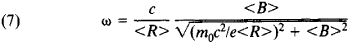This condition follows from equations (3) and (2). It can be seen from equation (7) that when the magnetic field increases the frequency of revolution initially increases in proportion to the field and then changes more and more slowly as it approaches the limiting value c/<R> corresponding to motion of the particle at the speed of light; the frequency of the accelerating field ωa = ωq must change accordingly. Since the radius of the equilibrium orbit is constant, the magnet of the proton synchrotron can be made in the form of a comparatively thin ring. The cost of the machine is thereby greatly reduced.

Among present-day accelerators, proton synchrotrons produce the highest particle energies. Until 1972 the largest accelerator in the world was the proton synchrotron near Serpukhov in the USSR; it can accelerate protons to an energy of 76 GeV. In 1972 a 200-GeV proton synchrotron was completed at Batavia in the USA. Its energy was increased to 400 GeV in 1975 and to 500 GeV in 1976. A 400-GeV accelerator went into operation in 1976 at the European Organization for Nuclear Research (CERN), near Geneva. Proton synchrotrons capable of reaching 1,000 GeV and even higher energies are under development.

Since the limiting value of the magnetic field is restricted by engineering capabilities, it follows from equation (2) that an increase in energy entails an increase in the radius of the machine. The radii of the accelerators in which the greatest energies have been achieved are hundreds of meters. Planned accelerators capable of reaching even higher energies will have radii of several kilometers. The size and, consequently, cost of an accelerator are the factors that limit the maximum attainable energy. Proton synchrotrons are not used to reach energies of less than approximately 1 GeV; to produce lower-energy protons it is advantageous to employ synchrocyclotrons (see below).

Protons are introduced, or injected, into a proton synchrotron from another, lower-energy accelerator. Such a preaccelerator may be a linear accelerator, or it may be a booster ring accelerator into which particles are injected from a linear accelerator. The increase in injection energy permitted by such a multistage scheme has several useful consequences. For example, the conditions for operation of the main accelerator are lightened: it is easier to maintain the tolerances for precise reproduction of the magnetic field during injection, and the range of variation of the accelerating-field frequency is smaller. Other consequences are a reduction in accelerator costs and an increase in the beam intensity achievable in the accelerator.

Weak-focusing proton synchrotron. In a weak-focusing proton synchrotron the magnetic system consists of several magnet sectors separated by straight sections (Figure 6). Injection systems, accelerating devices, beam-monitoring systems, vacuum pumps, and other equipment are located in the straight sections.

The injection system feeds particles from the injector, or pre-accelerator, into the vacuum chamber of the main accelerator. Injection is usually accomplished by means of a pulsed deflector whose electric or magnetic field bends the beam of injected particles and directs it into orbit.

The vacuum chamber is a continuous closed tube that envelops the region around the equilibrium orbit. Since the particles being accelerated may be scattered by the residual gas, the pressure must be sufficiently low that such scattering does not result in expansion of the beam and loss of particles. A vacuum of ~10–6mm Hg is produced in the chamber by means of continuously operating pumps.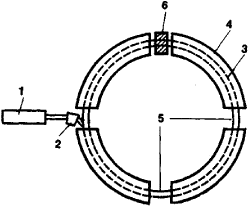Figure 6. Schematic of a weak-focusing electron or proton synchrotron: (1) injector, (2) injection system, (3) vacuum chamber, (4) electromagnet sector, (5) straight section, (6) accelerating device. The magnetic field is perpendicular to the plane of the figure.

The circular sections of the chamber are located in the gaps between the poles of the electromagnets that generate in the chamber the magnetic field needed to guide the motion of the particles in a closed orbit—that is, to bend the particle beam along the orbit. Since the radius of the equilibrium orbit must remain constant, the magnetic field must increase during acceleration from a value corresponding to the injection energy to a maximum value corresponding to the final energy. The increase in the magnetic field is accomplished by increasing the current flowing through the windings of the electromagnets. The shape of the magnet poles is selected so as to provide a small radial falloff of the magnetic field in accordance with condition (4). This falloff is necessary for stable motion of the particles in the transverse direction.

Accelerating devices that generate an alternating electric field are located in one or more straight sections. The field frequency varies strictly in accordance with the variation of the magnetic field [equation (7)]. The required precision of frequency reproduction is extremely great and is usually accomplished by means of an automatic frequency monitoring system using data on particle position. Since an error in frequency leads to departure of the particles from the equilibrium position, sensitive pickups are employed to register this departure, and their signal is amplified and used to make the required corrections in the frequency.

Under the action of the accelerating field the particles in the injected beam break up into bunches that group around stable equilibrium phases. The number of such bunches, which are located along the periphery of the accelerator, is equal to q. During acceleration the bunches become shorter in length by contracting toward the equilibrium phase. At the same time, a decrease occurs in the transverse dimensions of the beam, which at the start of acceleration occupies nearly the entire cross section of the vacuum chamber.

Alternating-gradient proton synchrotron. The alternating-gradient, or strong-focusing, proton synchrotron is distinguished above all by the arrangement of the magnet system, which consists of a large number of magnets in which a strong decrease and a strong increase in the magnetic field along the radius alternate. Particle focusing is much stronger in this case than in a weak-focusing accelerator. In one approach each magnet (Figure 7) performs the two functions of bending the particle beam in its orbit and focusing the beam. A separated-function design is also used. Magnets with uniform fields are used for bending, and focusing is accomplished by means of magnetic quadrupole lenses located in sections between the bending magnets.

The adoption of alternating-gradient magnetic systems entails more severe tolerances for magnet construction and mounting. For a ring magnet with a length greater than 1 km, the accuracy of alignment is measured in tenths or hundredths of a millimeter because of the high sensitivity of particle behavior to various random deviations of the magnetic field. In an inaccurately shaped field this sensitivity can lead to resonant buildup of particle oscillations.

Another characteristic of the alternating-gradient accelerator is the existence of a critical, or transition, energy. At a particle energy less than the critical energy, the stable equilibrium phase is located on the rising side of the voltage curve (phase – φ0 in Figure 1), since the period decreases with increasing energy, as in a linear accelerator. At a particle energy greater than the critical energy, by contrast, an increase in energy leads to an increase in the period revolution, as in a weak-focusing accelerator, and the phase + φ0 becomes the equilibrium phase. In order to prevent beam losses when the critical energy is passed, at the time of transition through the critical energy a rapid 2φ0 shift in the phase of the oscillations is introduced into the system, so that the particles being accelerated, which below the critical energy were grouped near the stable phase – φ0, end up in the region of the new stable phase + φ0.

A beam accelerated in a proton synchrotron may be fed to an internal target within the vacuum chamber. Alternatively, the beam may be extracted from the accelerator by means of a deflector that is of the same type used in the injection system but is more powerful because of the high particle speed. A new accelerating cycle then commences. The accelerating cycle repetition frequency in proton synchrotrons now in use is 5–30 cycles per minute. In each cycle 1011–1013 particles are accelerated. In principle the maximum intensity is determined by the limiting influence of the space charge.

Since a proton synchrotron capable of accelerating particles to very high energies requires very large dimensions and very severe tolerances in the construction of the machine (particularly the magnets), the feasibility of using superconducting materials in accelerator electromagnets and of employing methods of automatically controlling accelerator parameters is being explored. By using superconducting materials, magnetic fields at least three or four times greater than conventional fields can be obtained, and the size of the machine can be reduced by the same factor. By applying automatic control methods to accelerator parameters, the construction tolerances can be eased.Figure 7. Schematic cross section of a magnet of an alternating-gradient accelerator: (1) pole pieces shaped so as to provide a sharp change in the magnetic field along the radius, (2) electromagnet windings, (3) cross section of vacuum chamber, (B) magnetic field

ELECTRON SYNCHROTRON. The electron synchrotron is a circular resonance accelerator of electrons. The difference between the electron synchrotron and the proton synchrotron is that in the electron synchrotron only the magnetic field varies with time; the frequency of the accelerating electric field remains constant. At a constant frequency of revolution the orbit radius is proportional to the particle speed (R = v/ω); for electrons the speed becomes close to the speed of light at energies of the order of 1 MeV—that is, the speed changes little with increasing energy. Consequently, the radius of the equilibrium orbit in an electron synchrotron changes almost not at all. The magnet of the electron synchrotron, like that of the proton synchrotron, therefore has the form of a ring. Both weak- and strong-focusing electron synchrotrons are extremely similar to the proton synchrotron in design.

The maximum energies attainable in an electron synchrotron are determined primarily by the electromagnetic radiation of relativistic electrons. Since electrons moving in circular trajectories undergo centripetal acceleration, the laws of electrodynamics require that the electrons emit electromagnetic waves (seeSYNCHROTRON RADIATION). The energy emitted by an electron in one revolution is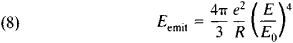where Ey = m0c2 is the rest energy of the particle, which for the electron is equal to 0.5 MeV. The energy emitted thus increases very rapidly with increasing electron energy. In large electron accelerators the energy emitted per revolution is comparable to the energy acquired by the particle. It may be noted that, in principle, electromagnetic radiation is generated when any charged particles move in a circle. For heavy particles (protons or nuclei), however, E0 is much greater than it is for electrons; consequently, heavy particles do not exhibit significant radiation at the energies achieved in accelerators.

The energy eV0 cos φ0 imparted to an electron by the accelerating field goes in part to increasing the particle energy and in part to radiation. The radiation affects particle oscillations about the equilibrium orbit. On the one hand, the radiation acts like friction in that it damps the oscillations of the particle. On the other hand, because the radiation is quantized and involves the emission of photons, the slowing down occurs not smoothly but in “clicks,” a circumstance that introduces additional buildup of oscillations.

Because of the large losses to radiation, the accelerating system must be of high power. Although the constant frequency of revolution makes possible the use of fixed-frequency resonant systems, the difficulty of building the accelerating system restricts the maximum attainable energies. By 1976 maximum energies of the order of 5–10 GeV had been reached in electron synchrotrons (see Table 3). Plans exist for electron synchrotrons capable of 100–150 GeV. In electron synchrotrons for lower energies (hundreds of millions of electron volts), betatron injection is often used instead of injection from an external preaccelerator, as in a proton synchrotron. When betatron injection is used, the accelerator initially operates as a betatron; after the electron reaches relativistic speeds (V ≈ c), the accelerating radio-frequency field is switched on, and the accelerator begins synchrotron operation.

SYNCHROCYCLOTRON. The synchrocyclotron, also known as the frequency-modulated cyclotron, is the third main type of circular resonance accelerator making use of the phase stability principle. In the synchrocyclotron the magnetic field is constant, and the frequency of the accelerating electric field varies; equation (3’) indicates that an increase in equilibrium energy requires a decrease in frequency. The synchrocyclotron is used to accelerate heavy particles (protons, deuterons, and alpha particles). The largest synchrocyclotrons now in use produce protons with a kinetic energy of up to 1,000 MeV.

In the synchrocyclotron particles move in spiral trajectories from the center, where a gas-discharge ion source is located, to the periphery of the vacuum chamber (Figure 8). They acquire energy through repeated passage across the accelerating gap. The accelerated particles either are used inside the vacuum chamber or are extracted to the outside by means of deflection systems. The accelerating-field frequency is changed by means of a variable capacitor in a resonant circuit. Because the particle orbit in a synchrocyclotron has a spiral shape, the synchrocyclotron requires a solid-core rather than ring magnet. As a result, the magnetic system is extremely bulky. Preference is therefore given to the proton synchrotron at energies above 1 GeV, although it produces accelerated beams of much lower intensity.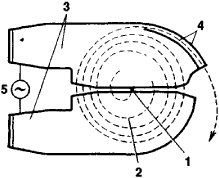Figure 8. Schematic of particle motion in the cyclotron and synchrocyclotron: (1) ion source, (2) spiral orbit of a particle undergoing acceleration, (3) accelerating electrodes, (4) extraction device (deflector plates), (5) source of accelerating field. The magnetic field is directed perpendicularly to the plane of the drawing.

In synchrocyclotrons with an azimuthally uniform magnetic field, vertical focusing is very weak, since n ≪ 1. For better vertical focusing, additional, azimuthal variations of the magnetic field are sometimes used—that is, alternating-gradient focusing is employed.

The three types of resonance accelerators described above, which make use of the phase stability principle, are characterized by pulsed operation: the group of particles bunched around the equilibrium phase increases its energy as the appropriate change

Table 3. Largest circular accelerators
Type and locationMaximum energy (GeV)Diameter of machine (m)Size of vacuum chamber (cm)Type of injectorEnergy at injection (MeV)Date of first operation
1As of 1976
Proton synchrotron
Dubna, USSR ...............107235 × 120Linac9.41957
Argonne, USA ...............12.75515 × 82Linac501963
Geneva, Switzerland ...............282007 × 15Linac501959
Booster8001972
Brookhaven, USA ...............332578 × 1 7Linac2001960
Serpukhov, USSR ...............7647212 × 20Linac1001967
Booster
Batavia, USA ...............50012,0005 × 13Booster8,0001972
Electron synchrotron
Daresbury, Great Britain ...............5.270(4–6) × (11–15)Linac431966
Yerevan, USSR ...............6.1693 × 1 0Linac501967
Hamburg, Federal Republic of Germany ...............7.5101(4–7) × (10–12)Linac300–5001964
Cornell University, Ithaca, USA ...............12.22502.5 × 5.5Linac1501967
Synchrocyclotron
Geneva, Switzerland ...............0.605.01957
Dubna, USSR ...............0.686.01953
Leningrad, USSR ...............1.006.851968

in the accelerating-field frequency and/or magnetic induction occurs. After maximum energy is reached, the group of particles either is used inside the vacuum chamber or is extracted from the accelerator. The accelerator parameters then revert to their initial values, and a new accelerating cycle begins. The duration of the acceleration pulse is of the order of hundredths of a second in electron synchrotrons and synchrocyclotrons and of the order of a few seconds in proton synchrotrons.

CYCLOTRON. The cyclotron is a circular resonance accelerator for protons or ions. In this machine both the magnetic field and the frequency of the accelerating electric field are constant. Unlike accelerators described previously, the cyclotron provides continuous output. It is similar in design to the synchrocyclotron.Figure 9. Schematic cross section of a betatron: (1) magnet poles, (2) cross section of annular vacuum chamber, (3) central core, (4) electromagnet windings, (5) magnet yoke

In a cyclotron particles from the ion source continuously enter the vacuum chamber, where they are accelerated by electrodes and move in a spiral. The quantities ωa and B do not vary with time in a constant-gradient cyclotron, but the particle energy increases. Consequently, resonance condition (3’) is violated, and resonance acceleration can occur only as long as the kinetic energy W acquired is much less than the rest energy m0c2—that is, only until the relativistic increase in the particle’s mass begins to manifest itself. This fact determines the upper limit of the energies attainable in a cyclotron; for protons the limit is approximately 10–20 MeV. The limiting energy is achieved at very high voltages on the accelerating electrodes. On the other hand, because of its continuous operation, the cyclotron provides a high beam intensity. The magnetic field in a cyclotron has a very small radial falloff; a large falloff of the field, it may be noted, would intensify still further the deviation from exact resonance. Magnetic-field focusing in the vertical direction is therefore very weak (n ≈ 0), especially at the center of the magnet. In the central region, however, the particle speeds are still small, and electric-field focusing has a substantial influence.

Exact resonance between a particle and the constant-frequency accelerating field could be maintained in a cyclotron if the magnetic field increased with radius. In a constant-gradient accelerator such an increase is impermissible because of the resulting instability of motion in the vertical direction. If, however, alternating-gradient focusing is used, stable acceleration can be achieved to much higher energies than in ordinary cyclotrons. Machines of this type are called isochronous, or sector, cyclotrons. They have the same advantage of high intensity as cyclotrons have and are capable of producing intense proton beams at energies of up to 1,000 MeV. The isochronous cyclotron at the Swiss Institute for Nuclear Research delivers a proton current of 12 microamperes (µ A); the maximum energy of the accelerated particles in the cyclotron is 590 MeV.

MICROTRON. The microtron, or electron cyclotron, is a circular resonance accelerator in which, as in the cyclotron, both the magnetic field and the accelerating-field frequency are constant in time. In a microtron, however, resonance is maintained during acceleration through a variation in q.

A particle revolves in a microtron in a uniform magnetic field and repeatedly passes through an accelerating cavity. The particle’s energy gain in the cavity is such that the particle’s period of revolution changes by an amount that is equal to or a multiple of the accelerating-voltage period. If the particle revolves in resonance with the accelerating field from the very start, this resonance is maintained despite the change in the period of revolution. Suppose, for example, the particle makes its first revolution in one period of the accelerating field (that is, q = 1), its second revolution in two periods (q = 2), its third revolution in three periods (q = 3), and so on. Clearly, the particle always crosses the accelerating cavity at the same phase. A type of phase stability exists in the microtron, so that near-equilibrium particles are also accelerated.

The microtron produces a continuous output. Some machines are capable of delivering currents of the order of 100 milliamperes (mA). The maximum energy reached is of the order of 30 MeV, which has been attained in machines in the USSR and Great Britain. It is difficult to reach higher energies because of the severe magnetic-field tolerances required; substantial increases in current are limited by the synchrotron radiation of the electrons being accelerated.

For prolonged maintenance of resonance, the magnetic field of a microtron must be uniform. Since such a field does not have vertical focusing properties, the focusing is accomplished with the electric field of the accelerating cavity. Modifications of the microtron have been proposed with an azimuthally varying magnetic field (the sector microtron), but they have not undergone serious development.

BETATRON. The betatron, which is used to accelerate electrons, is the only nonresonance-type circular accelerator.

Electrons are accelerated in the betatron by means of the vorticity electric field induced by time-varying magnetic flux passing through the core, or central part, of a magnet. An annular vacuum chamber is located in the gap between the magnet poles. The pole faces are specially shaped so as to impart a small radial falloff to the magnetic field; the field causes revolution of the particles in a circle and provides focusing of the particles with respect to the average equilibrium radius (Figure 9). In order for the orbit radius to remain constant, there must exist a definite relation, called the betatron condition, between the rate of energy increase, which is determined by the change in the field in the central part, and the rate of increase of the magnetic guide field. The relation reduces to the condition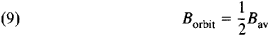which means that the field at the orbit (Borbit) should be half the average field (Bav) within the orbit. When this condition and focusing condition (4) are satisfied, stable acceleration of particles occurs in an orbit of constant radius.

The betatron has a pulsed output and can be used as a source of electrons of energies of the order of 100–300 MeV. For energies above 100–200 MeV, however, the electron synchrotron, which does not have a bulky central core, is more convenient. Betatrons for energies of 20–50 MeV are particularly common; they are used for various purposes and are lot produced. As noted above, betatron-type acceleration is often used in electron synchrotrons for preacceleration. Since such acceleration is to a low energy, the core required for betatron operation is small and does not substantially complicate the design of the synchrotron.

Linear accelerators, ELECTROSTATIC ACCELERATOR. Electrostatic accelerators are discussed in ACCELERATOR, HIGH-VOLTAGE.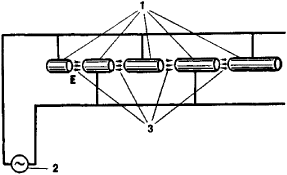Figure 10. Schematic of a Wideröe-type linear accelerator: (1) drift tubes, (2) source of alternating voltage, (3) region of action of electric field E

LINEAR INDUCTION ACCELERATOR. In a linear induction accelerator particles are accelerated by the electromotive force induced upon a change in a ring-shaped magnetic field. Ferromagnetic rings surrounded by current windings are mounted along the accelerator axis. A sharp change in the current in the windings causes a rapid change in the magnetic field. In accordance with the law of electromagnetic induction, this change in the magnetic field generates an electric field E at the accelerator axis. A charged particle traveling along the axis during the existence of this field acquires an energy eEL, where L is the distance traversed. For the accelerating field to be sufficiently great, the magnetic field must be changed rapidly. The lifetime of the accelerating field and, consequently, the duration of the acceleration pulse are therefore small (of the order of 10–9–10–6 sec).

The advantages of linear induction accelerators are large accelerated-particle currents (hundreds or thousands of amperes), high beam uniformity (a small energy spread and small velocities of transverse motion), and high efficiency of conversion of power consumed by the accelerating system into output beam power. Existing linear induction accelerators produce electron beams having an energy of a few million electron volts and are used principally as sources of intense relativistic-electron beams in collective acceleration machines and in devices for research on thermonuclear fusion. The linear induction accelerator has, however, potentially much broader application.

LINEAR RESONANCE ACCELERATORS. Linear resonance machines constitute the most widely used type of linear accelerator, especially at high energies. Linear resonance accelerators for electrons produce energies ranging from tens of millions of electron volts to ~20 GeV; machines for protons produce energies of up to 800 MeV. The substantial difference in energy between proton and electron linear accelerators is due chiefly to the circumstance that protons are accelerated to nonrelativistic or slightly relativistic speeds, whereas electrons can be accelerated to very high relativistic speeds. Proton linear accelerators designed for energies of ~600–800 MeV, at which relativistic effects become appreciable, approach electron linear accelerators in design. The parameters of the largest linear accelerators are given in Table 4.

Proton linear resonance accelerator. The idea of the linear resonance accelerator was advanced in 1924 by the Swedish scientist G. Ising and was implemented by Wideröe in 1928. This type of accelerator (Figure 10) consists of a system of hollow cylinders, called drift tubes, connected alternately to opposite poles of an alternating-voltage source. The electric field does not penetrate the tubes and is concentrated in the gaps between them. The lengths of the tubes are such that if particles enter the first gap between tubes at a time when the field is directed so as to accelerate the particles, they will also enter subsequent gaps at an accelerating phase of the field. Thus, the particles’ energy will continually increase, and we may speak of resonance acceleration. An accelerator of approximately this type was built in 1931 by Lawrence and D. Sloan of the USA.

Advances in high-frequency radio engineering in the 1940’s gave further impetus to the development of proton linear accelerators. Instead of lumped-constant circuits, modern proton accelerators generally use the scheme proposed by the American physicist L. Alvarez, which involves a resonant cavity with drift tubes. Within the cavity, which is of cylindrical shape, an alternating electric field directed along the cavity’s axis is generated. The particles being accelerated pass through the system of drift tubes in such a way that they cross the accelerating gaps between tubes at times when the field is directed with the particle motion (Figure 11). When the field is directed in the opposite direction, the particles are located inside the tubes, which the field cannot penetrate.

A linear resonance accelerator, as indicated above, makes use of phase stability, so that the particles located in some region about the equilibrium particle are accelerated together with it and, on the average, acquire the same energy. The stable equilibrium phase in a linear accelerator is negative—that is, is located in the interval where the field rises. The electric field therefore has a defocusing action in a linear accelerator, and special steps must be taken to provide focusing of the protons. In low-energy accelerators foil or grid focusing may be employed: the input apertures of the drift tubes are covered with a foil or grid barrier. This approach deforms the field between tubes, and the defocusing region vanishes almost entirely. In high-energy accelerators this focusing method cannot be used, since the foils and grids cause intolerable losses of intensity; moreover, the foils and grids burn up under the action of the beam. The most widely used focusing method is alternating-gradient focusing with magnetic quadrupole lenses located inside the drift tubes. The lenses produce in the vicinity of the accelerator axis a magnetic field that increasesFigure 11. Schematic cross section of the Alvarez linear accelerator structure: (1) cavity, (2) drift tubes. Near the axis the electric field E is found only in the gaps between tubes.

linearly with distance from the axis. Qualitatively, focusing by means of such a field can be explained in the same way as for circular accelerators.

The advantages of linear accelerators over circular accelerators include the absence of a cumbersome magnetic system, simplicity of particle injection and extraction, and high current densities. The capabilities of proton linear accelerators are limited, however, by the complexity and high cost of the radio-engineering system required and by the difficulty of focusing. At the present time proton linear accelerators are used primarily as injectors for ring accelerators. The injectors reach an energy of 50–100 MeV or even 200 MeV. At higher energies the Alvarez structure becomes inefficient from a radio-engineering standpoint, since too much power is required in order to produce the electric field (the shunt impedance is too low). Special systems of coupled cavities have been developed for acceleration to higher energies. A waveguide system with diaphragms may also be used, as in electron linear accelerators.

Table 4. Largest linear accelerators
Type and locationDate of first operationMaximum energy (MeV)Length (m)Duration of pulse of accelerated particles (μ sec)Maximum average current (μA)Maximum current in pulse (mA)
Electron linear accelerator Kharkov, USSR ...............19641,8002401.40.8
Stanford University, Palo Alto, USA ...............196622,3003,0501.648
Proton linear accelerator
Serpukhov, USSR (injector) ...............196710080300 180
Batavia, USA (injector) ...............1970200145400 120
Los Alamos, USA (meson factory) ...............19728007955001,000
Academy of Sciences, USSR (meson factory) ...............Under construction600450100500

Present-day high-energy linear accelerators of protons consist of two stages: in the first stage, acceleration is carried out to 100–200 MeV with Alvarez-type cavities; the second stage has cavities of different type whose characteristics are more favorable at such particle energies. This two-stage scheme is used in the 800-MeV proton linear accelerator at Los Alamos in the USA, which can produce an average current of 1,000 µA. The facility is used for physics experiments with intense secondary beams and is sometimes referred to as a meson factory. A 600-MeV meson factory using the same scheme is under construction in the USSR.

Electron linear resonance accelerator. Electron linear resonance accelerators have an additional advantage over circular accelerators: because the velocity of the electrons is practically constant in both magnitude and direction, electrons in linear accelerators emit almost no radiation. The maximum energy reached by present-day electron linear accelerators is ~20 GeV, but this upper limit is dictated solely by economic considerations and could be raised by simply increasing the length.

For electron linear accelerators, in which particles travel almost from the very beginning at a speed close to that of light, the most advantageous accelerating system is a traveling-wave structure in the form of a diaphragm-loaded wave guide. In a wave guide with smooth walls, electromagnetic waves travel with a phase speed that exceeds the speed of light. In order for a traveling wave to be able to accelerate particles, it must move with the same speed as the particles—that is, to accelerate electrons, the wave must be slowed down to the speed of light. This slowing down can be accomplished by, for example, introducing diaphragms into the wave guide (Figure 12).Figure 12. Schematic cross section of a diaphragm-loaded wave guide: (1) diaphragms, (2) electron bunch undergoing acceleration. The arrows indicate the configuration of the field traveling along the wave guide.

Since the electron speed is close to the speed of light, the motion of the electrons with respect to the accelerating waves exhibits special characteristics. For example, the phase stability principle does not apply to the electrons: a change in the energy of an electron causes almost no change in its speed and, consequently, does not lead to a shift relative to the accelerating wave. Focusing in the transverse direction is also generally unnecessary, because the speeds of the electrons’ random transverse motions decrease as the electrons’ energy increases. (The law of conservation of momentum requires that the transverse momentum mv remain constant; moreover, according to the theory of relativity, the mass m increases with increasing energy. Consequently, the transverse speed v decreases.) Furthermore, transverse Coulomb repulsion in electron accelerators is almost canceled by the magnetic attraction of parallel currents. The electron bunches being accelerated can, however, excite in the accelerating wave guide spurious waves that lead to beam blowup. This effect is of particular importance in large accelerators, where it limits the maximum attainable currents. A number of engineering methods of suppressing the effect have been developed.

Electron linear accelerators operating at low energies of the order of tens of millions of electron volts are widely encountered. The accelerators are used for research in nuclear and neutron physics and have many practical applications.

Intensive studies are being carried out on the feasibility of using superconducting materials for the walls of cavities and wave guides in proton and electron linear accelerators. Such materials would greatly reduce the consumption of microwave power and would make possible continuous, rather than pulsed, operation of linear accelerators.

Accelerators for particles other than electrons and protons. The type of particle accelerators described above can be used to accelerate not only electrons and protons but also other charged particles. With practically no modifications electron accelerators can be employed to accelerate positrons. Various types of proton accelerators are used to accelerate heavy particles. The highest ion energy has been achieved in the Bevalac accelerator in the USA. This machine, which is based on a proton synchrotron, in 1974 accelerated nuclei as heavy as the argon nucleus to an energy of 2 GeV per nucleon. At Dubna plans have been drawn up for an accelerator, called a nuklotron, that would be capable of reaching 16 GeV per nucleon. Linear accelerators and cyclotron-type accelerators are also used as sources of heavy ions.

### REFERENCES

Grinberg, A. P. Metody uskoreniia zariazhennykh chastits. Moscow-Leningrad, 1950.
Uskoriteli (collection of articles). Moscow, 1962. (Translated from English and German.)
Kolomenskii, A. A. and A. N. Lebedev. Teoriia tsiklicheskikh uskoritelei. Moscow, 1962.
Bruck, H. Tsiklicheskie uskoriteli zariazhennykh chastits. Moscow, 1970. (Translated from French.)
Val’dner, O. A., A. D. Vlasov, and A. V. Shal’nov. Lineinye uskoriteli. Moscow, 1969.
Komar, E. G. Osnovy uskoritel’noi tekhniki. Moscow, 1975.
Sokolov, A. A., and I. M. Ternov. Reliativistskii elektron. Moscow, 1974.

E. L. BURSHTEIN

Site: Follow: Share:
Open / Close